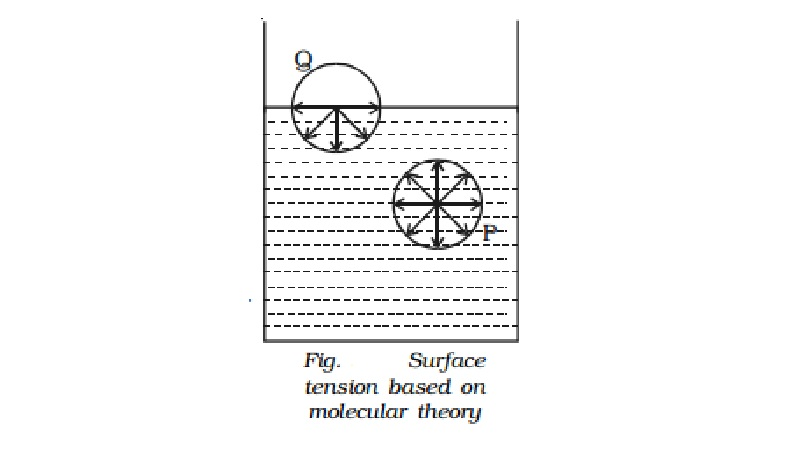Home | | Physics | | Physics | Molecular theory of surface tension

# Molecular theory of surface tensionConsider two molecules P and Q as shown in Fig.. Taking them as centres and molecular range as radius, a sphere of influence is drawn around them.

Molecular theory of surface tension

Consider two molecules P and Q as shown in Fig.. Taking them as centres and molecular range as radius, a sphere of influence is drawn around them.

The molecule P is attracted in all directions equally by neighbouring molecules. Therefore net force acting on P is zero. The molecule Q is on the free surface of the liquid. It experiences a net downward force because the number of molecules in the lower half of the sphere is more and the upper half is completely outside the surface of the liquid. Therefore all the molecules lying on the surface of a liquid experience only a net downward force.

If a molecule from the interior is to be brought to the surface of the liquid, work must be done against this downward force. This work done on the molecule is stored as potential energy. For equilibrium, a system must possess minimum potential energy. So, the free surface will have minimum potential energy. The free surface of a liquid tends to assume minimum surface area by contracting and remains in a state of tension like a stretched elastic membrane.

Surface tension of a liquid

Surface tension is the property of the free surface of a liquid at rest to behave like a stretched membrane in order to acquire minimum surface area.

Imagine a line AB in the free surface of a liquid at rest (Fig.). The force of surface tension is measured as the force acting per unit length on either side of this imaginary line AB. The force is perpendicular to the line and tangential to the liquid surface. If F is the force acting on the length l of the line AB, then surface tension is given by T=F/l.

Surface tension is defined as the force per unit length acting perpendicular on an imaginary line drawn on the liquid surface, tending to pull the surface apart along the line. Its unit is N m-1 and dimensional

formula is MT-2.

Study Material, Lecturing Notes, Assignment, Reference, Wiki description explanation, brief detail

Related Topics